# Telangana SSC Board Question Paper for Class 10th General Science Part I 2016 In PDF

## Telangana 10th Standard General Science Part I Exam Question Paper 2016 with Solutions – Free Download

Telangana Board Class 10 General Science Part I 2016 Question Paper with Solutions is the best resource for the important questions, some of which are likely to be repeated in the General Science Part I Physical Sciences exams. Students can find the PDFs of the solved or unsolved question paper, as per preference. They can download these papers with just a click of the interactive links that are listed below. We have mentioned the interactive PDF links from where students can access the printable format of the question papers. The questions and answers are also published on the web-page.

The students who mastered the entire complex topics of Class 10 Physical Sciences will find it easy to ace the exams. They get familiar with the type of questions found in the TS Board SSC Class 10 General Science Part I exam by referring to these question paper solutions. Students are also advised to practise hard and solve the previous papers of Telangana Board Class 10 before the exams. Doing so is likely to help them self gauge their performance and preparation level for the board exams. It is also the best way to identify the mistakes and also take steps to avoid these mistakes in the board exams. Solving these paper solutions will help the students get an overview of the question paper design and the marks’ distribution per section. These question paper solutions also help them to scale the difficulty level of the exams.

### Telangana Board Class 10 General Science Part I 2016 Question Paper with Solutions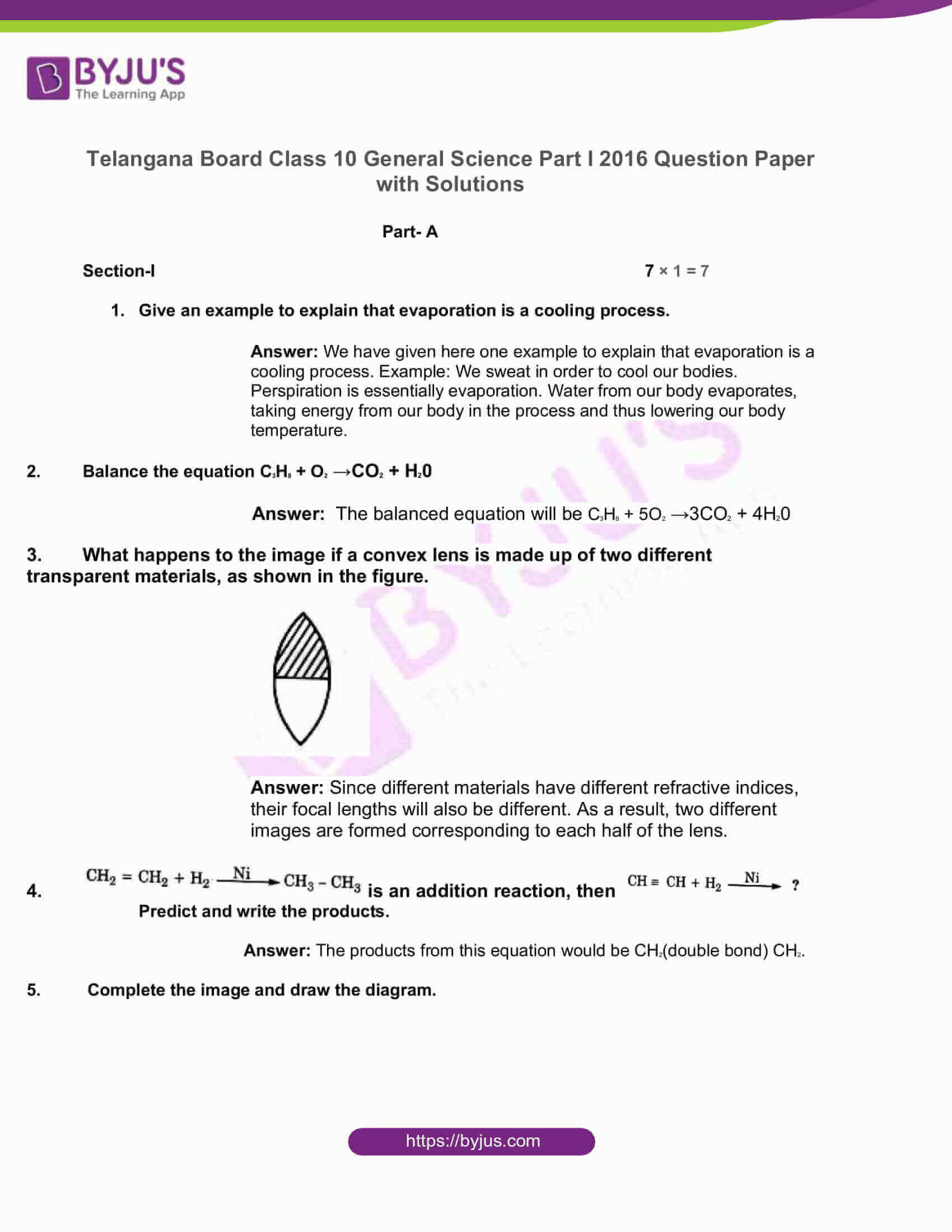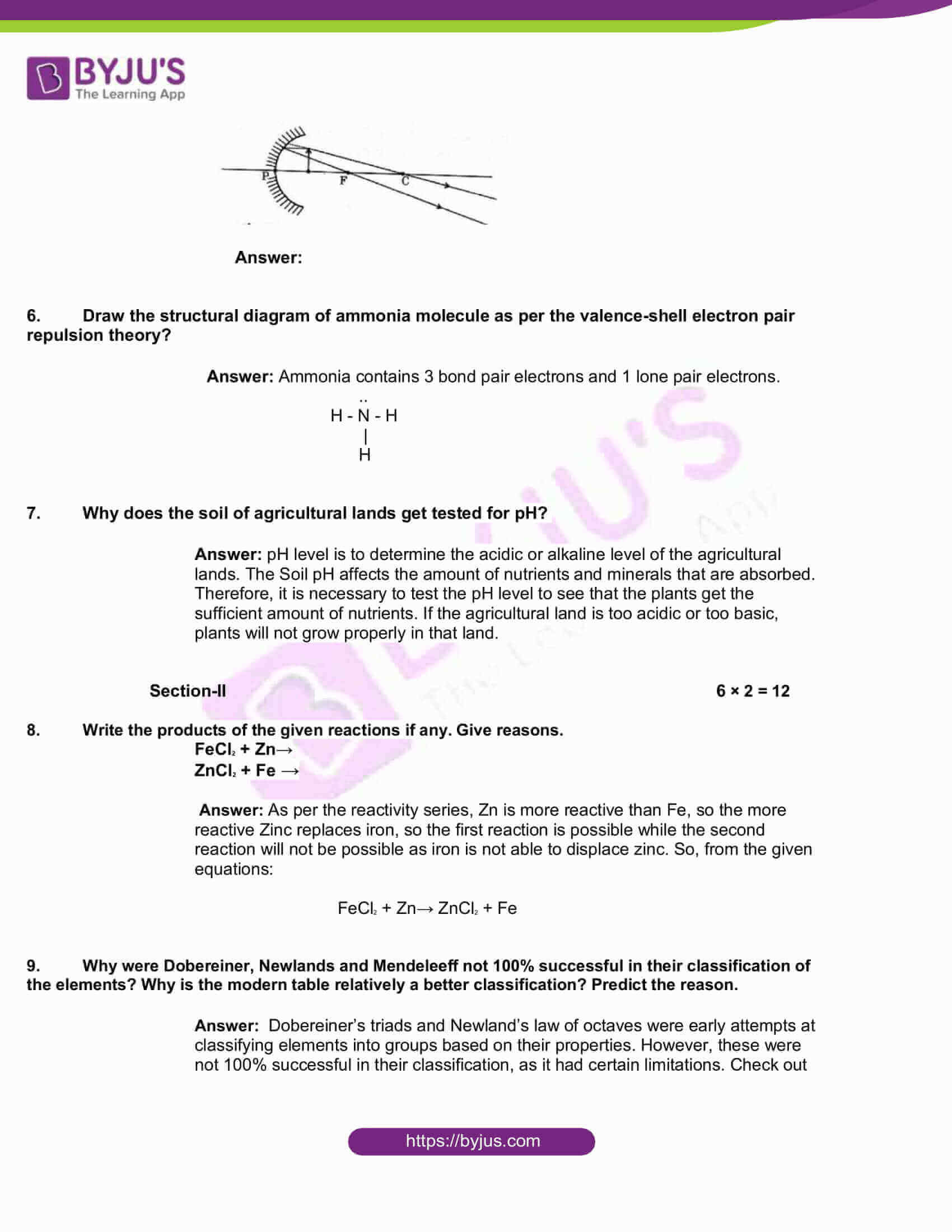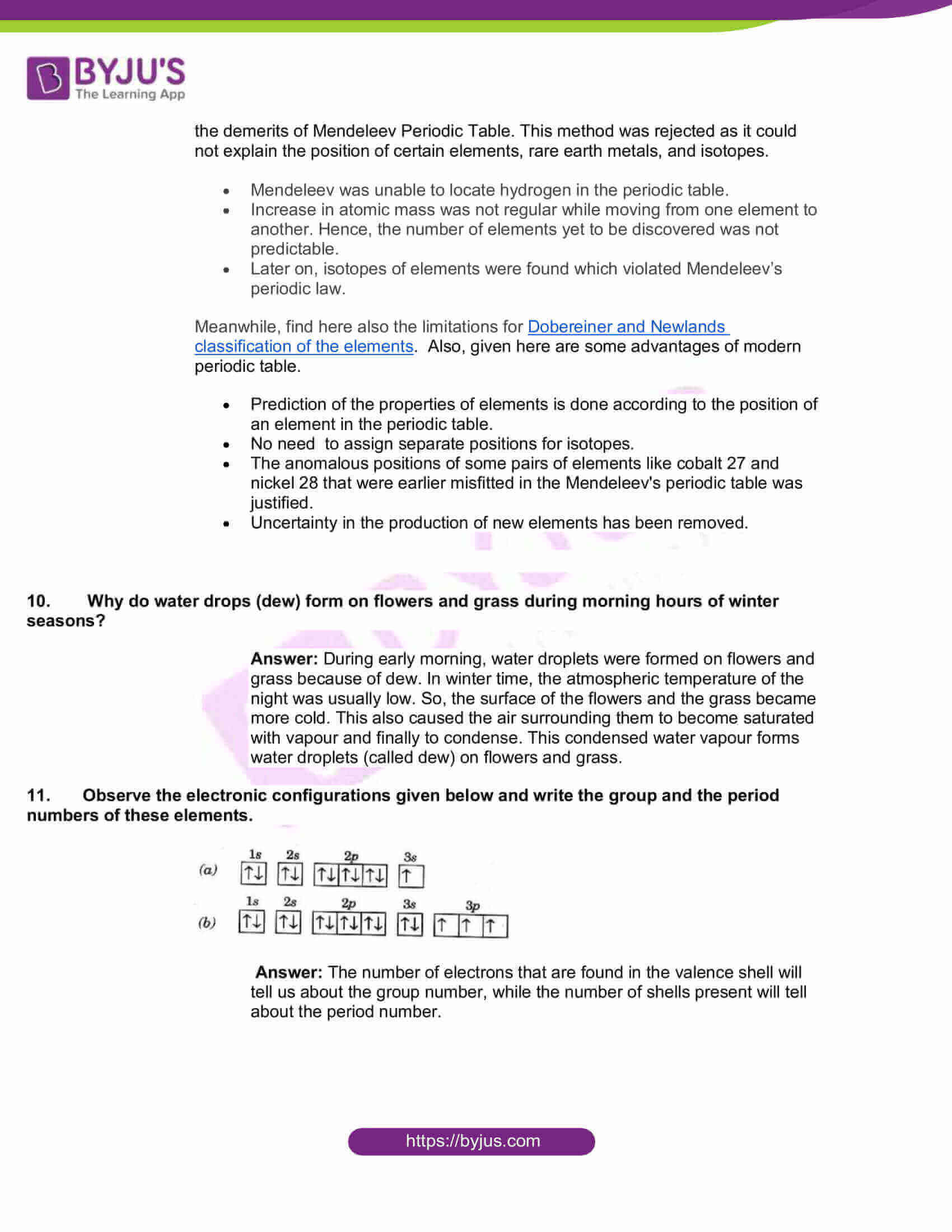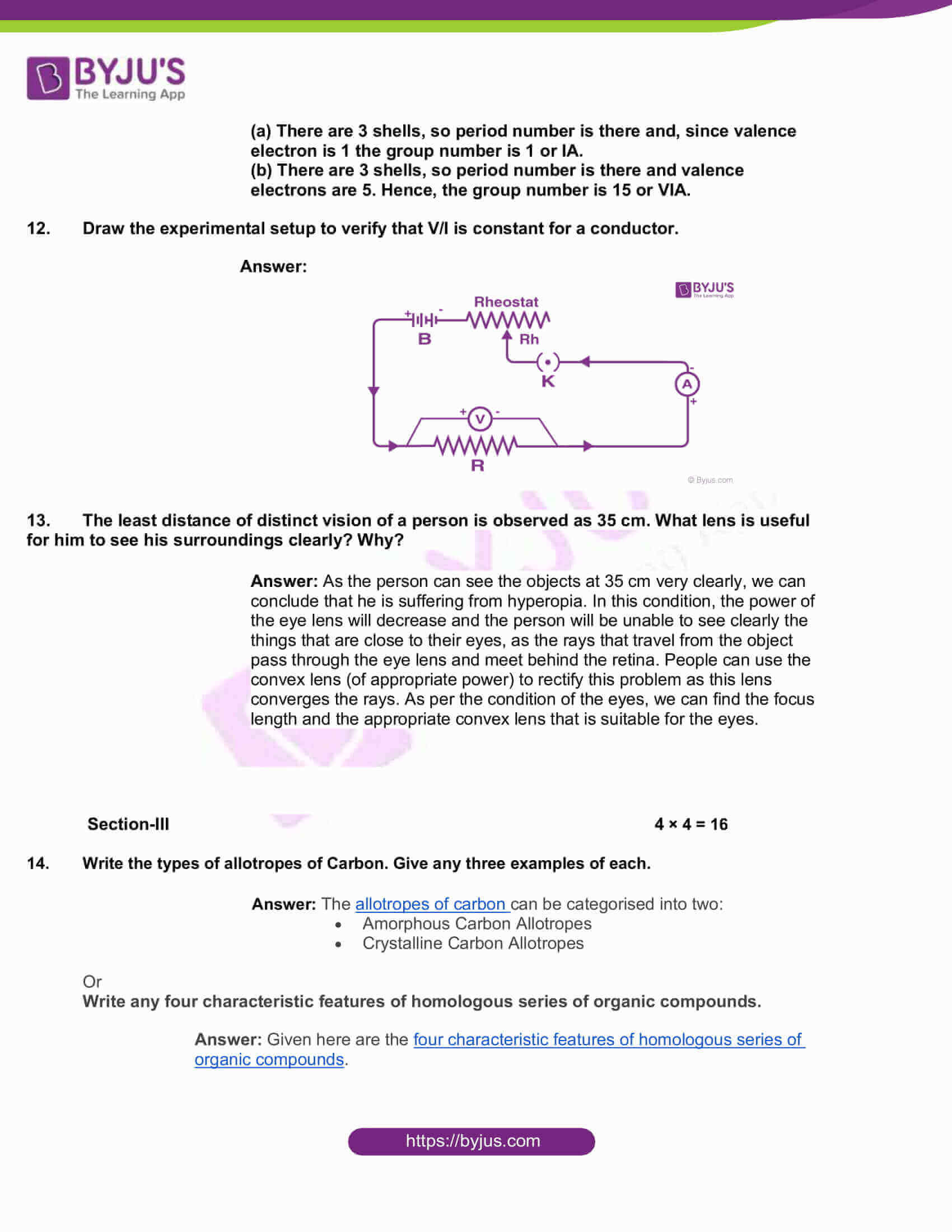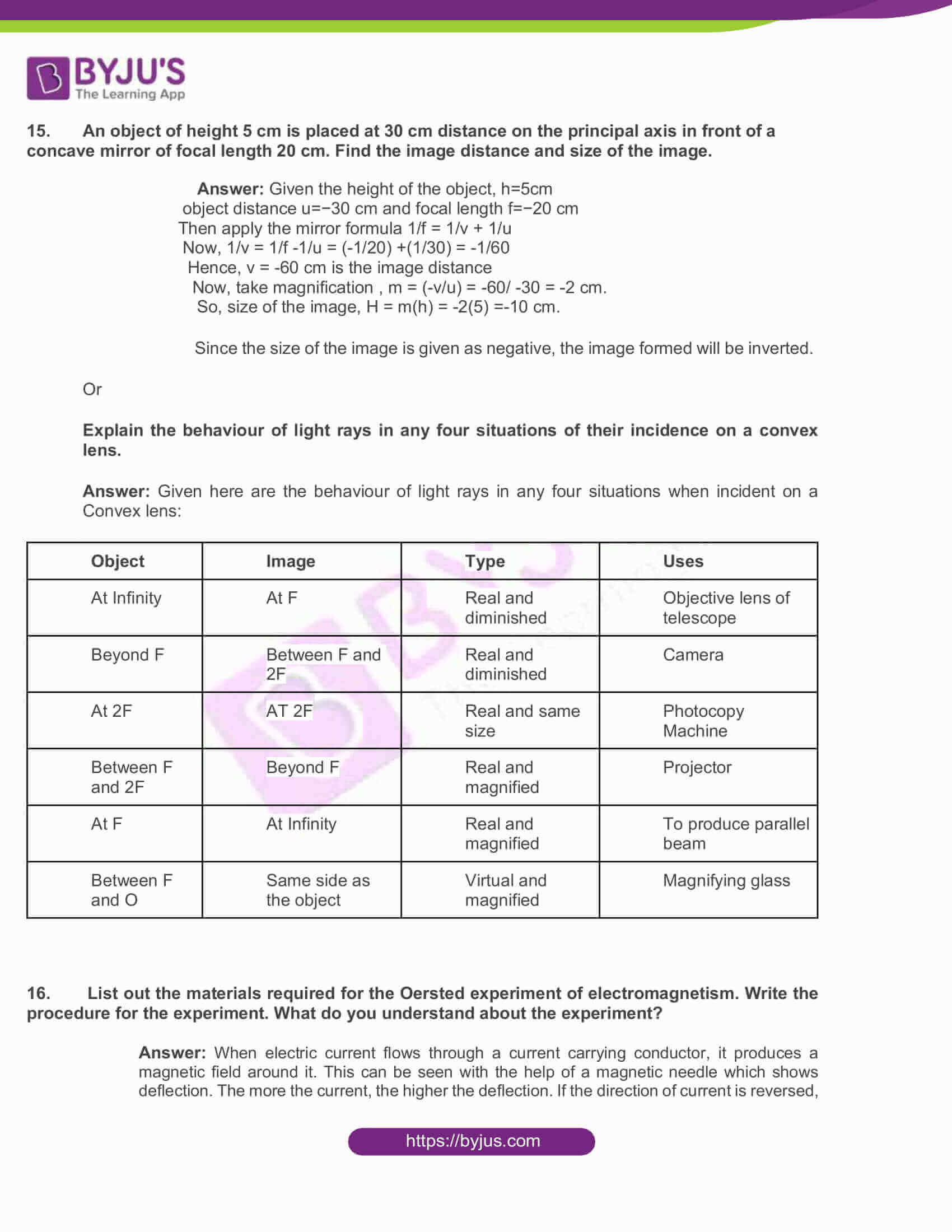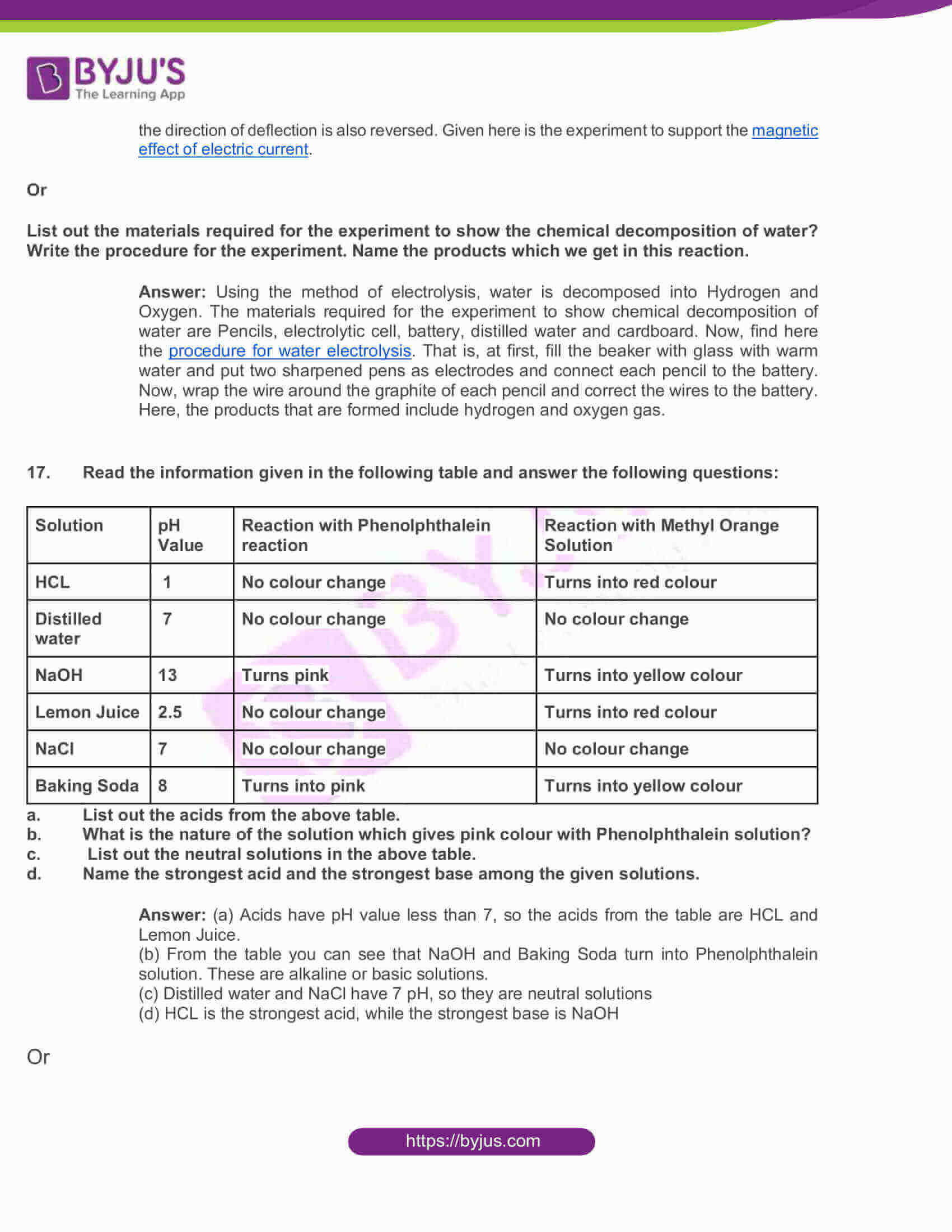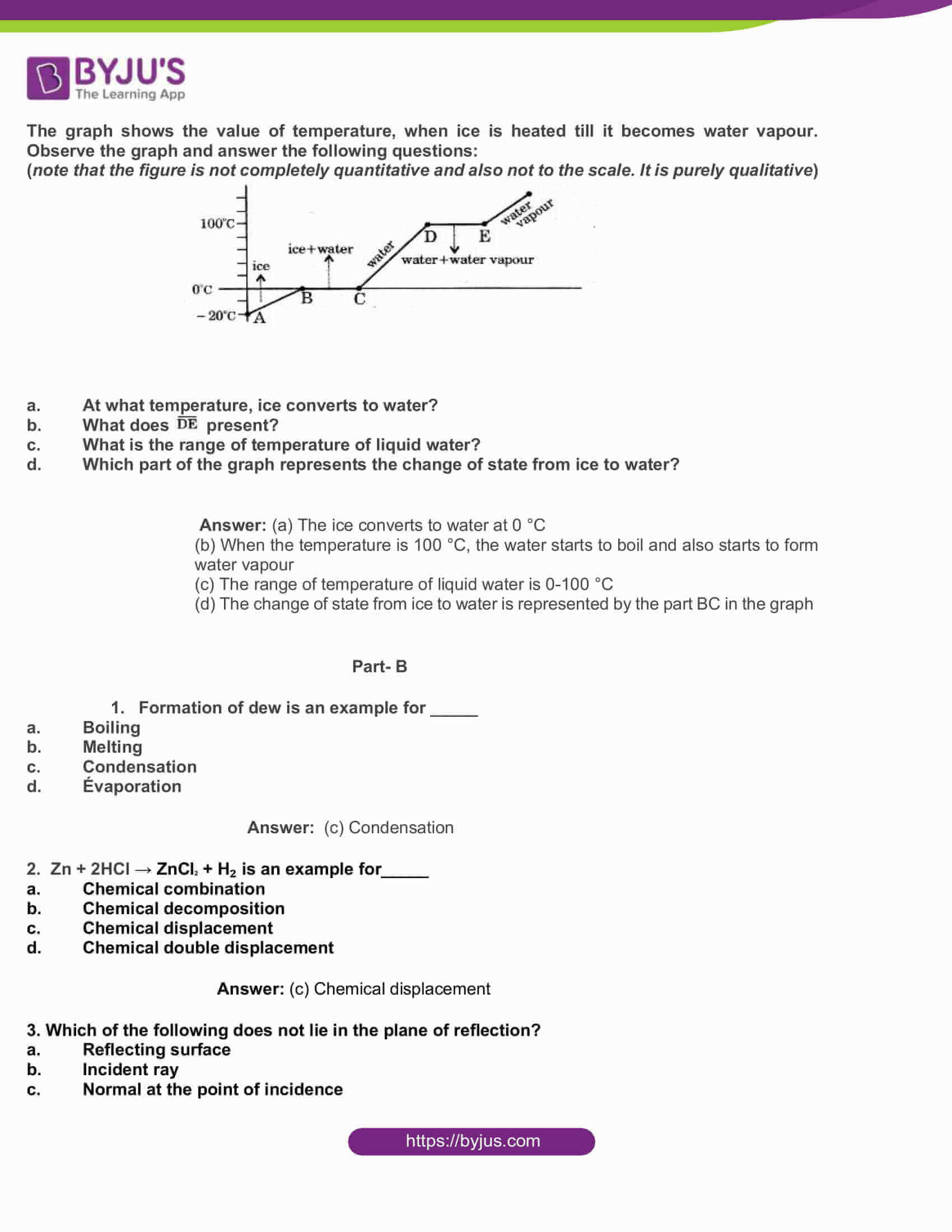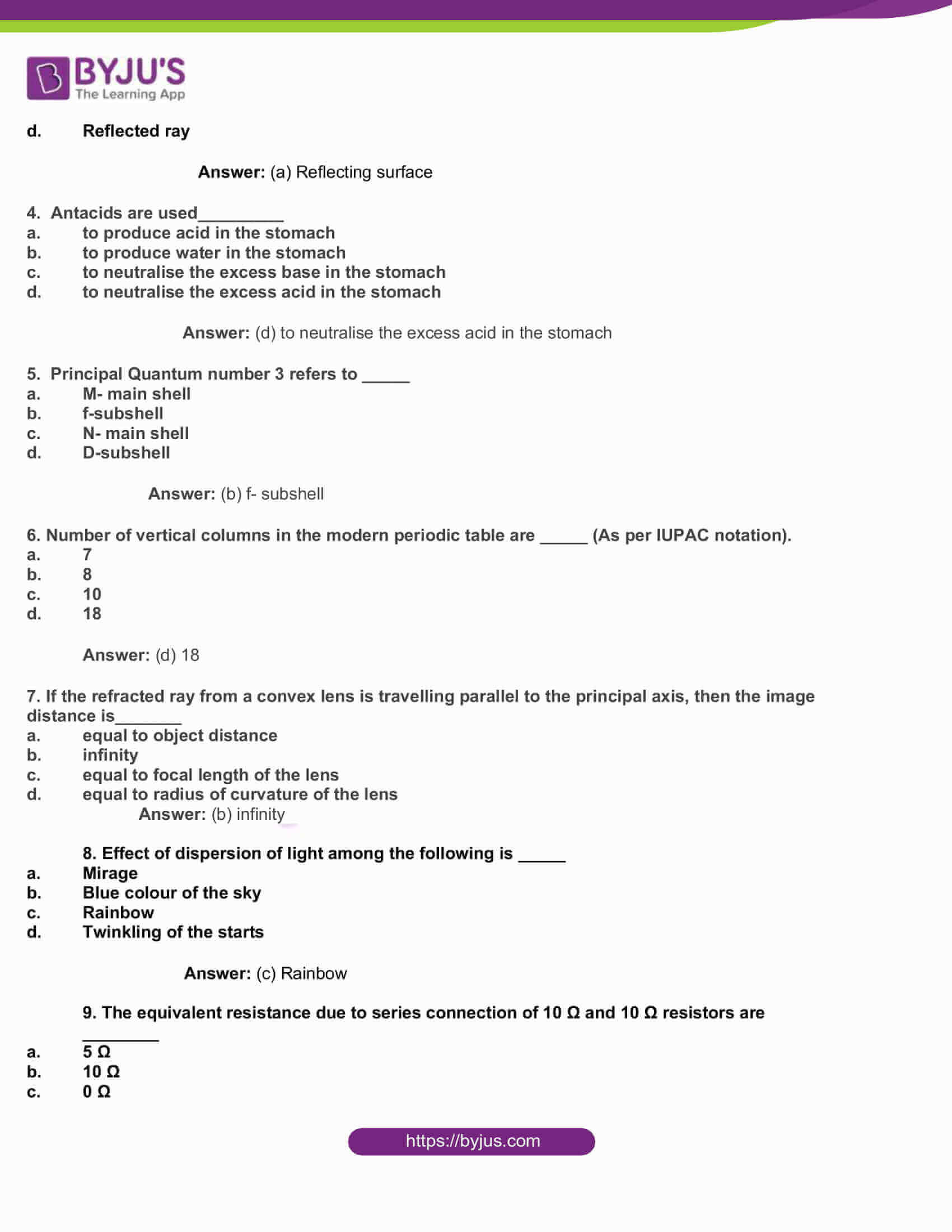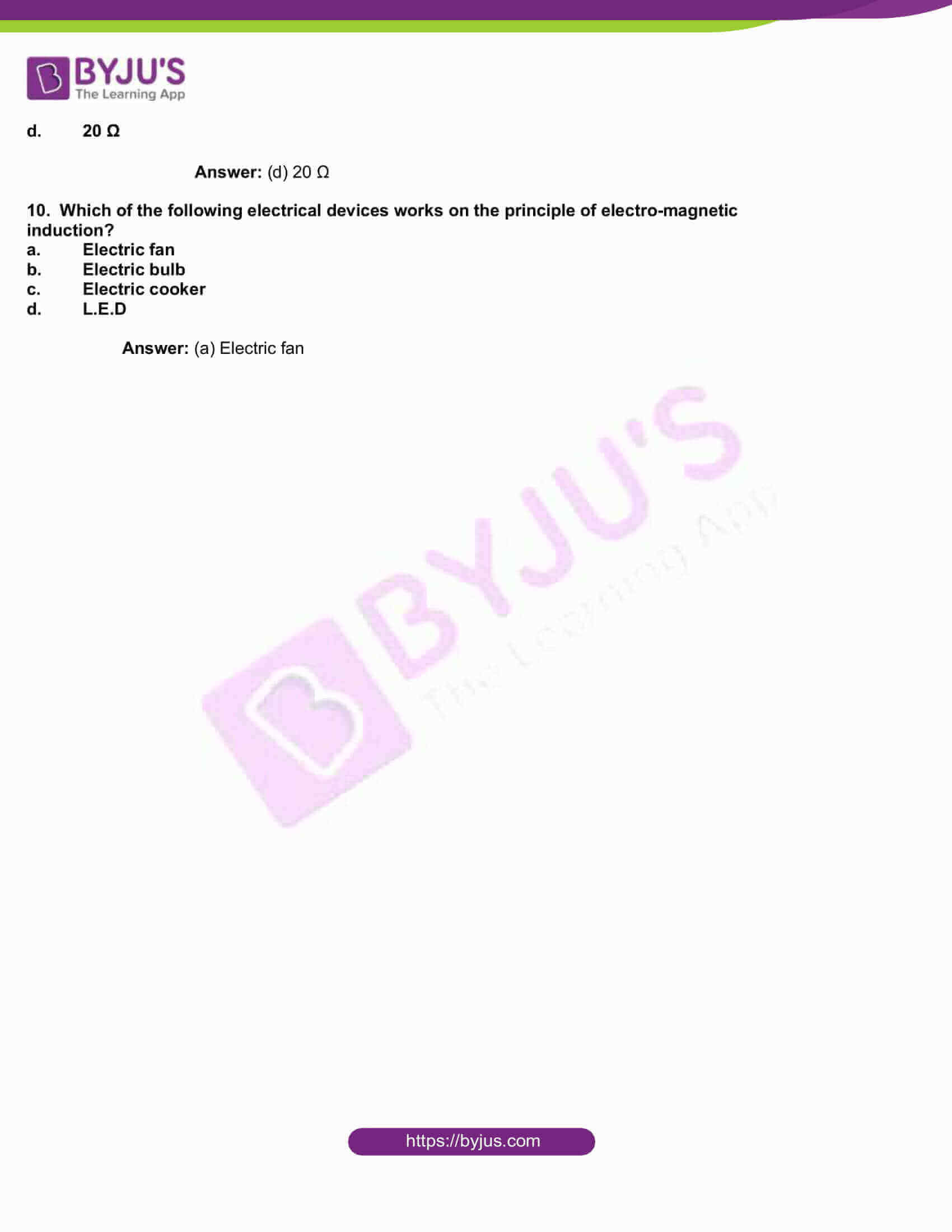Part- A

Section-I 7 × 1 = 7

1. Give an example to explain that evaporation is a cooling process.

Answer: We have given here one example to explain that evaporation is a cooling process. Example: We sweat in order to cool our bodies. Perspiration is essentially evaporation. Water from our body evaporates, taking energy from our body in the process and thus lowering our body temperature.

1. Balance the equation C3H8 + O2 →CO2 + H20

Answer: The balanced equation will be C3H8 + 5O2 →3CO2 + 4H20

1. What happens to the image if a convex lens is made up of two different transparent materials, as shown in the figure.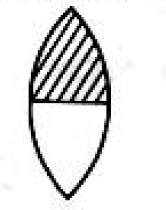Answer: Since different materials have different refractive indices, their focal lengths will also be different. As a result, two different images are formed corresponding to each half of the lens.

1.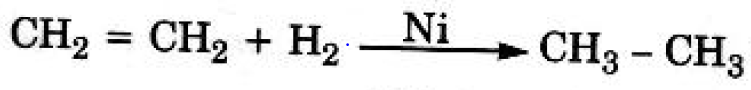is an addition reaction, then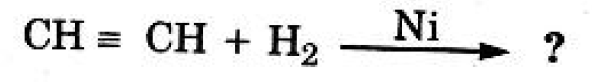Predict and write the products.

Answer: The products from this equation would be CH2(double bond) CH2.

1. Complete the image and draw the diagram.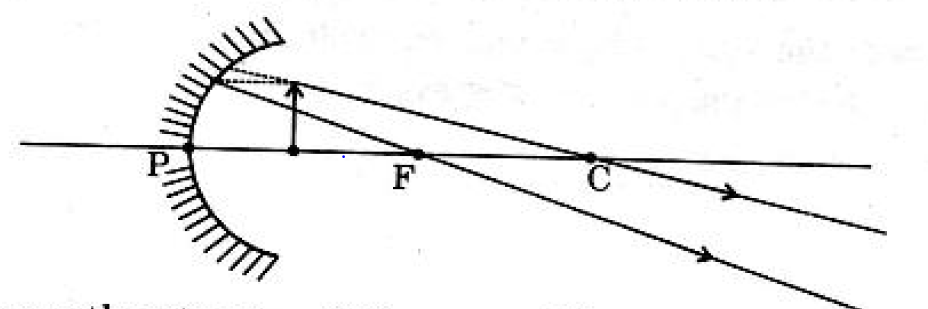1. Draw the structural diagram of ammonia molecule as per the valence-shell electron pair repulsion theory?

Answer: Ammonia contains 3 bond pair electrons and 1 lone pair electrons.

..

H – N – H

|

H

1. Why does the soil of agricultural lands get tested for pH?

Answer: pH level is to determine the acidic or alkaline level of the agricultural lands. The Soil pH affects the amount of nutrients and minerals that are absorbed. Therefore, it is necessary to test the pH level to see that the plants get the sufficient amount of nutrients. If the agricultural land is too acidic or too basic, plants will not grow properly in that land.

Section-II 6 × 2 = 12

1. Write the products of the given reactions if any. Give reasons.

FeCl2 + Zn

ZnCl2 + Fe →

Answer: As per the reactivity series, Zn is more reactive than Fe, so the more reactive Zinc replaces iron, so the first reaction is possible while the second reaction will not be possible as iron is not able to displace zinc. So, from the given equations:

FeCl2 + Zn→ ZnCl2 + Fe

1. Why were Dobereiner, Newlands and Mendeleeff not 100% successful in their classification of the elements? Why is the modern table relatively a better classification? Predict the reason.

Answer: Dobereiner’s triads and Newland’s law of octaves were early attempts at classifying elements into groups based on their properties. However, these were not 100% successful in their classification, as it had certain limitations. Check out the demerits of Mendeleev Periodic Table. This method was rejected as it could not explain the position of certain elements, rare earth metals, and isotopes.

• Mendeleev was unable to locate hydrogen in the periodic table.
• Increase in atomic mass was not regular while moving from one element to another. Hence, the number of elements yet to be discovered was not predictable.
• Later on, isotopes of elements were found which violated Mendeleev’s periodic law.

Meanwhile, find here also the limitations for Dobereiner and Newlands classification of the elements. Also, given here are some advantages of modern periodic table.

• Prediction of the properties of elements is done according to the position of an element in the periodic table.
• No need to assign separate positions for isotopes.
• The anomalous positions of some pairs of elements like cobalt 27 and nickel 28 that were earlier misfitted in the Mendeleev’s periodic table was justified.
• Uncertainty in the production of new elements has been removed.
1. Why do water drops (dew) form on flowers and grass during morning hours of winter seasons?

Answer: During early morning, water droplets were formed on flowers and grass because of dew. In winter time, the atmospheric temperature of the night was usually low. So, the surface of the flowers and the grass became more cold. This also caused the air surrounding them to become saturated with vapour and finally to condense. This condensed water vapour forms water droplets (called dew) on flowers and grass.

1. Observe the electronic configurations given below and write the group and the period numbers of these elements.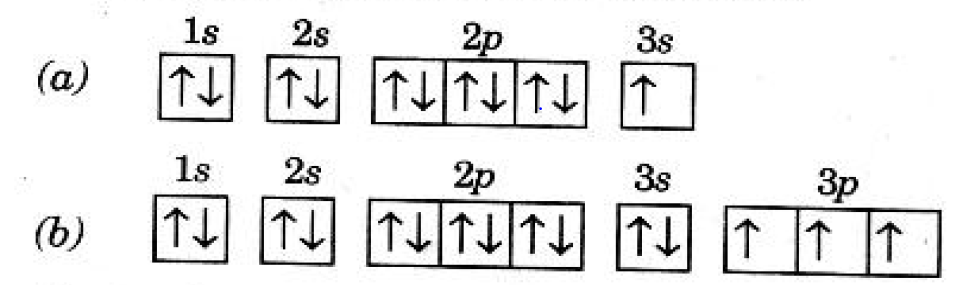Answer: The number of electrons that are found in the valence shell will tell us about the group number, while the number of shells present will tell about the period number.

## (b) There are 3 shells, so period number is there and valence electrons are 5. Hence, the group number is 15 or VIA.

1. Draw the experimental setup to verify that V/I is constant for a conductor.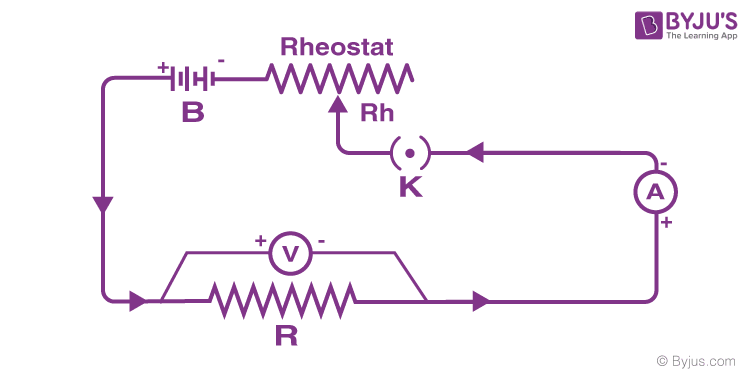1. The least distance of distinct vision of a person is observed as 35 cm. What lens is useful for him to see his surroundings clearly? Why?

Answer: As the person can see the objects at 35 cm very clearly, we can conclude that he is suffering from hyperopia. In this condition, the power of the eye lens will decrease and the person will be unable to see clearly the things that are close to their eyes, as the rays that travel from the object pass through the eye lens and meet behind the retina. People can use the convex lens (of appropriate power) to rectify this problem as this lens converges the rays. As per the condition of the eyes, we can find the focus length and the appropriate convex lens that is suitable for the eyes.

Section-III 4 × 4 = 16

1. Write the types of allotropes of Carbon. Give any three examples of each.

Answer: The allotropes of carbon can be categorised into two:

• Amorphous Carbon Allotropes
• Crystalline Carbon Allotropes

Or

Write any four characteristic features of homologous series of organic compounds.

Answer: Given here are the four characteristic features of homologous series of organic compounds.

1. An object of height 5 cm is placed at 30 cm distance on the principal axis in front of a concave mirror of focal length 20 cm. Find the image distance and size of the image.

Answer: Given the height of the object, h=5cm

object distance u=−30 cm and focal length f=−20 cm

Then apply the mirror formula 1/f = 1/v + 1/u

Now, 1/v = 1/f -1/u = (-1/20) +(1/30) = -1/60

Hence, v = -60 cm is the image distance

Now, take magnification , m = (-v/u) = -60/ -30 = -2 cm.

So, size of the image, H = m(h) = -2(5) =-10 cm.

Since the size of the image is given as negative, the image formed will be inverted.

Or

Explain the behaviour of light rays in any four situations of their incidence on a convex lens.

Answer: Given here are the behaviour of light rays in any four situations when incident on a Convex lens:

 Object Image Type Uses At Infinity At F Real and diminished Objective lens of telescope Beyond F Between F and 2F Real and diminished Camera At 2F AT 2F Real and same size Photocopy Machine Between F and 2F Beyond F Real and magnified Projector At F At Infinity Real and magnified To produce parallel beam Between F and O Same side as the object Virtual and magnified Magnifying glass
1. List out the materials required for the Oersted experiment of electromagnetism. Write the procedure for the experiment. What do you understand about the experiment?

Answer: When electric current flows through a current carrying conductor, it produces a magnetic field around it. This can be seen with the help of a magnetic needle which shows deflection. The more the current, the higher the deflection. If the direction of current is reversed, the direction of deflection is also reversed. Given here is the experiment to support the magnetic effect of electric current.

Or

List out the materials required for the experiment to show the chemical decomposition of water? Write the procedure for the experiment. Name the products which we get in this reaction.

Answer: Using the method of electrolysis, water is decomposed into Hydrogen and Oxygen. The materials required for the experiment to show chemical decomposition of water are Pencils, electrolytic cell, battery, distilled water and cardboard. Now, find here the procedure for water electrolysis. That is, at first, fill the beaker with glass with warm water and put two sharpened pens as electrodes and connect each pencil to the battery. Now, wrap the wire around the graphite of each pencil and correct the wires to the battery. Here, the products that are formed include hydrogen and oxygen gas.

1. Read the information given in the following table and answer the following questions:
 Solution pH Value Reaction with Phenolphthalein reaction Reaction with Methyl Orange Solution HCL 1 No colour change Turns into red colour Distilled water 7 No colour change No colour change NaOH 13 Turns pink Turns into yellow colour Lemon Juice 2.5 No colour change Turns into red colour NaCl 7 No colour change No colour change Baking Soda 8 Turns into pink Turns into yellow colour
1. List out the acids from the above table.
2. What is the nature of the solution which gives pink colour with Phenolphthalein solution?
3. List out the neutral solutions in the above table.
4. Name the strongest acid and the strongest base among the given solutions.

Answer: (a) Acids have pH value less than 7, so the acids from the table are HCL and Lemon Juice.

(b) From the table you can see that NaOH and Baking Soda turn into Phenolphthalein solution. These are alkaline or basic solutions.

(c) Distilled water and NaCl have 7 pH, so they are neutral solutions

(d) HCL is the strongest acid, while the strongest base is NaOH

Or

The graph shows the value of temperature, when ice is heated till it becomes water vapour. Observe the graph and answer the following questions:

(note that the figure is not completely quantitative and also not to the scale. It is purely qualitative)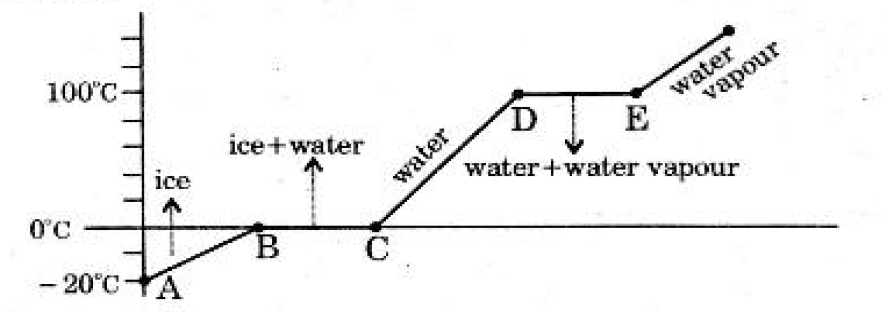1. At what temperature, ice converts to water?
2. What does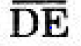present?
3. What is the range of temperature of liquid water?
4. Which part of the graph represents the change of state from ice to water?

Answer: (a) The ice converts to water at 0 °C

(b) When the temperature is 100 °C, the water starts to boil and also starts to form water vapour

(c) The range of temperature of liquid water is 0-100 °C

(d) The change of state from ice to water is represented by the part BC in the graph

Part- B

1. Formation of dew is an example for _____
2. Boiling
3. Melting
4. Condensation
5. Évaporation

2. Zn + 2HCl → ZnCl2 + H₂ is an example for_____

1. Chemical combination
2. Chemical decomposition
3. Chemical displacement
4. Chemical double displacement

3. Which of the following does not lie in the plane of reflection?

1. Reflecting surface
2. Incident ray
3. Normal at the point of incidence
4. Reflected ray

4. Antacids are used_________

1. to produce acid in the stomach
2. to produce water in the stomach
3. to neutralise the excess base in the stomach
4. to neutralise the excess acid in the stomach

Answer: (d) to neutralise the excess acid in the stomach

5. Principal Quantum number 3 refers to _____

1. M- main shell
2. f-subshell
3. N- main shell
4. D-subshell

6. Number of vertical columns in the modern periodic table are _____ (As per IUPAC notation).

1. 7
2. 8
3. 10
4. 18

7. If the refracted ray from a convex lens is travelling parallel to the principal axis, then the image distance is_______

1. equal to object distance
2. infinity
3. equal to focal length of the lens
4. equal to radius of curvature of the lens

8. Effect of dispersion of light among the following is _____

1. Mirage
2. Blue colour of the sky
3. Rainbow
4. Twinkling of the starts

9. The equivalent resistance due to series connection of 10 Ω and 10 Ω resistors are ________

1. 5 Ω
2. 10 Ω
3. 0 Ω
4. 20 Ω